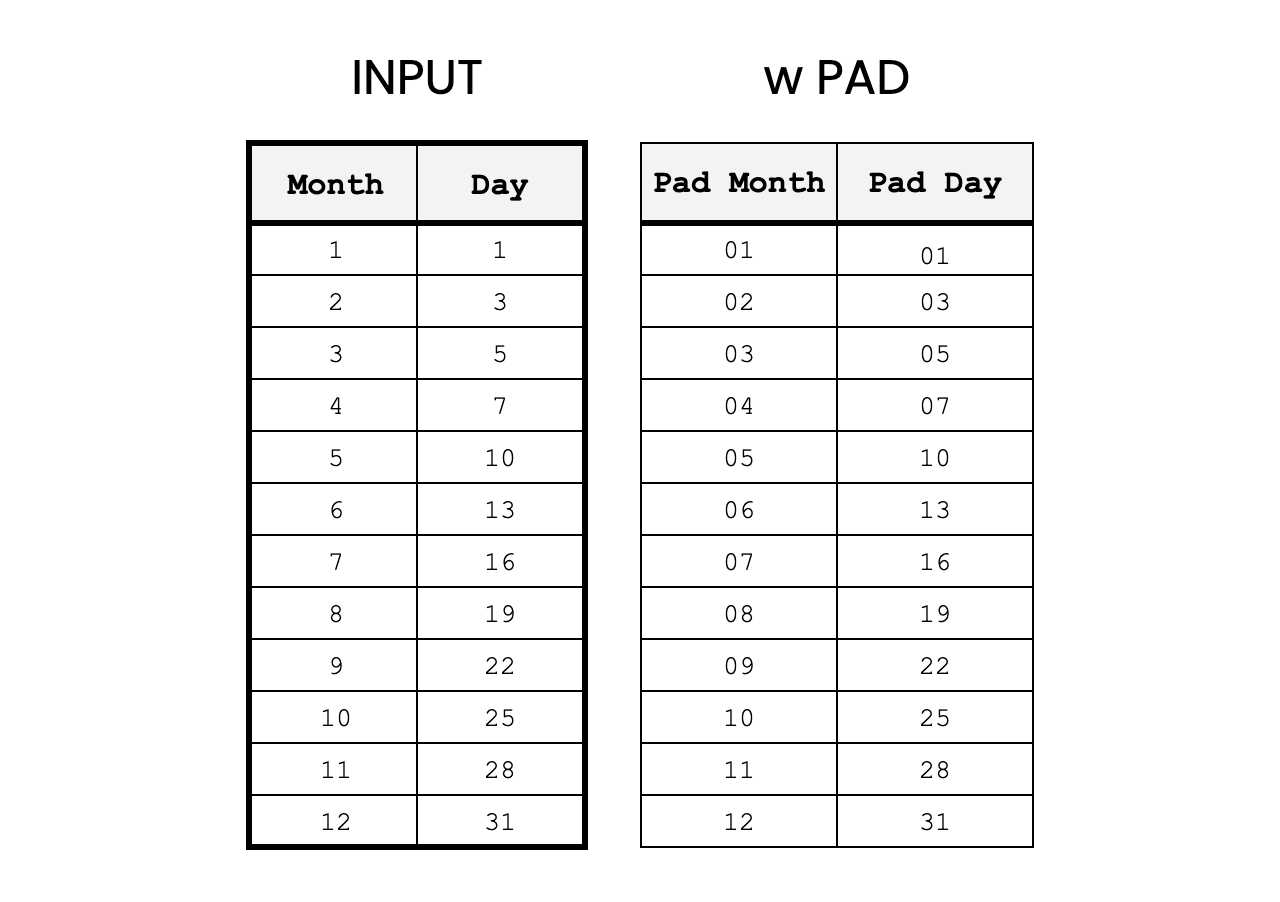Jul 7

The PAD function is a tool for creating output that all has the same length.

Commonly, this can be used to "pad" a single digit number with a leading zero so "1" becomes "01". This function is extensible so the user can decide what character to use and what the length should be. By example, it would be possible to turn "1" into "******1", as well.

### Function Signature

`=PAD(input, pad_length, pad_character)`

### Argument Definitions

input [range]

• required - range of cells to transform with padding.

• Number of characters that must be padded. Longer length strings will not be truncated.

• Function uses the first character of the given string as the padding character. Ex. if pad_character is "*", input is 1, the return would be "*1"

## Use the Cheat

Click the Code Box to Copy

Cheat copied to clipboard ✂️👍
``` /** * Pad's input with a "0" by default but can be used * for any replacement string. * * @param {all} input - any input that can be converted to string * * @param {number} pad_length - number of characters for output string. * Will add as many pad_characters as needed to reach length. * * @param {string} pad_character - the character to pad, by default it's "0" * * @return creates a string that's padded by the pad_character if the input is less than the * pad_length. If the pad_length is shorter than the input string - the input is returned, as is. * The return may be longer than "pad_length" if the input is longer. * * @customfunction */ function PAD(input, pad_length = 2, pad_character = "0") { const is_array = input instanceof Array; let ret = [[]]; if (input instanceof Array) { const is_matrix = input.length > 1; for (let i=0; i < input.length; i++) { if (is_matrix) { for (let j=0; j < input[i].length; j++) { if (!ret[i]) { ret[i] = []; } ret[i].push(single_pad_(input[i][j], pad_length, pad_character)); } } else { if (!ret[i]) { ret[i] = []; } ret[i].push(single_pad_(input[i], pad_length, pad_character)); } } } else { ret = [single_pad_(input, pad_length, pad_character)]; } return ret; } function single_pad_(input, pad_length, pad_character) { if (input === undefined || input.toString().length >= pad_length) { return input; } let final_string = input.toString(); final_string = final_string.padStart(pad_length, pad_character.toString()); return final_string; } ```Cyrus Radfar